# Run a Custom Training Experiment for Image Comparison

Since R2021a

This example shows how to create a custom training experiment to train a Siamese network that identifies similar images of handwritten characters. For a custom training experiment, you explicitly define the training procedure used by Experiment Manager. In this example, you implement a custom training loop to train a Siamese network, a type of deep learning network that uses two or more identical subnetworks that have the same architecture and share the same parameters and weights. Some common applications for Siamese networks include facial recognition, signature verification, and paraphrase identification.

This diagram illustrates the Siamese network architecture in this example.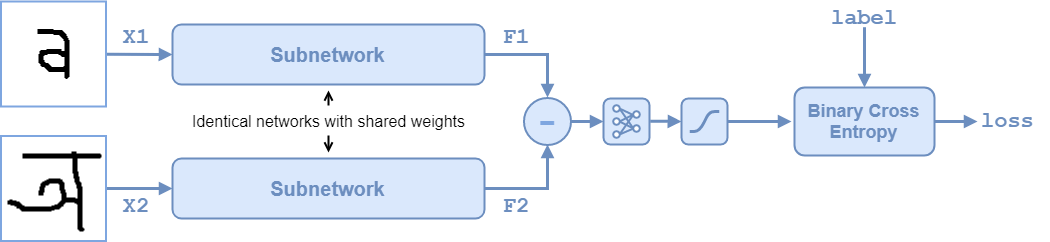To compare two images, you pass each image through one of two identical subnetworks that share weights. The subnetworks convert each 105-by-105-by-1 image to a 4096-dimensional feature vector. Images of the same class have similar 4096-dimensional representations. The output feature vectors from each subnetwork are combined through subtraction and the result is passed through a `fullyconnect` operation with a single output. A sigmoid operation converts this value to a probability indicating that the images are similar (when the probability is close to 1) or dissimilar (when the probability is close to 0). The binary cross-entropy loss between the network prediction and the true label updates the network during training. For more information, see Train a Siamese Network to Compare Images.

### Open Experiment

First, open the example. Experiment Manager loads a project with a preconfigured experiment that you can inspect and run. To open the experiment, in the Experiment Browser pane, double-click `ImageComparisonExperiment`.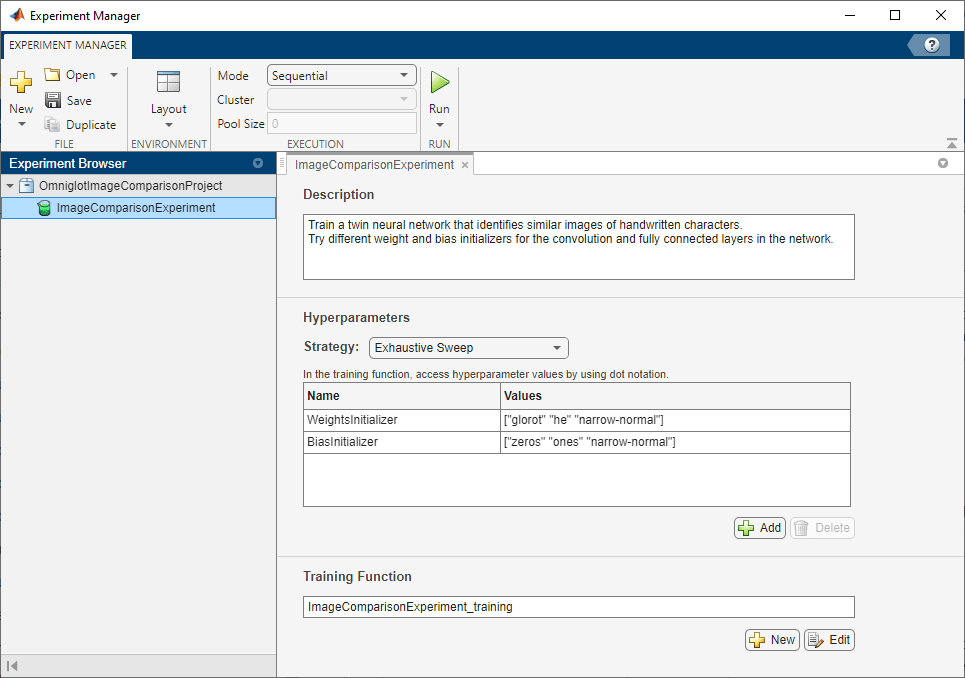Custom training experiments consist of a description, a table of hyperparameters, and a training function. For more information, see Configure Custom Training Experiment.

The Description field contains a textual description of the experiment. For this example, the description is:

```Train a Siamese network to identify similar and dissimilar images of handwritten characters. Try different weight and bias initializers for the convolution and fully connected layers in the network.```

The Hyperparameters section specifies the strategy and hyperparameter values to use for the experiment. When you run the experiment, Experiment Manager trains the network using every combination of hyperparameter values specified in the hyperparameter table. This example uses the hyperparameters `WeightsInitializer` and `BiasInitializer` to specify the weight and bias initializers, respectively, for the convolution and fully connected layers in each subnetwork. For more information about these initializers, see `WeightsInitializer` and `BiasInitializer`.

The Training Function section specifies a function that defines the training data, network architecture, training options, and training procedure used by the experiment. To open this function in MATLAB® Editor, click Edit. The code for the function also appears in Training Function. The input to the training function is a structure with fields from the hyperparameter table and an `experiments.Monitor` object that you can use to track the progress of the training, record values of the metrics used by the training, and produce training plots. The function returns a structure that contains the trained network, the weights for the final `fullyconnect` operation for the network, and the execution environment used for training. Experiment Manager saves this output so you can export it to the MATLAB workspace when the training is complete. The training function has these sections:

• Initialize Output sets the initial value of the network and `fullyconnect` weights to empty arrays to indicate that the training has not started. The experiment sets the execution environment to `"auto"`, so it trains and validates the network on a GPU if one is available. Using a GPU requires Parallel Computing Toolbox™ and a supported GPU device. For more information, see GPU Computing Requirements (Parallel Computing Toolbox).

```output.network = []; output.weights = []; output.executionEnvironment = "auto"; ```
• Load and Preprocess Training and Test Data defines the training and test data for the experiment as `imageDatastore` objects. The experiment uses the Omniglot data set, which consists of character sets for 50 alphabets, divided into 30 sets for training and 20 sets for testing. For more information on this data set, see Image Data Sets.

```monitor.Status = "Loading Training Data"; url = "https://github.com/brendenlake/omniglot/raw/master/python/images_background.zip"; downloadFolder = tempdir; filename = fullfile(downloadFolder,"images_background.zip"); dataFolderTrain = fullfile(downloadFolder,"images_background"); if ~exist(dataFolderTrain,"dir") websave(filename,url); unzip(filename,downloadFolder); end imdsTrain = imageDatastore(dataFolderTrain, ... IncludeSubfolders=true, ... LabelSource="none"); files = imdsTrain.Files; parts = split(files,filesep); labels = join(parts(:,(end-2):(end-1)),"_"); imdsTrain.Labels = categorical(labels); monitor.Status = "Loading Test Data"; url = "https://github.com/brendenlake/omniglot/raw/master/python/images_evaluation.zip"; filename = fullfile(downloadFolder,"images_evaluation.zip"); dataFolderTest = fullfile(downloadFolder,"images_evaluation"); if ~exist(dataFolderTest,"dir") websave(filename,url); unzip(filename,downloadFolder); end imdsTest = imageDatastore(dataFolderTest, ... IncludeSubfolders=true, ... LabelSource="none"); files = imdsTest.Files; parts = split(files,filesep); labels = join(parts(:,(end-2):(end-1)),"_"); imdsTest.Labels = categorical(labels); ```
• Define Network Architecture defines the architecture for two identical subnetworks that accept 105-by-105-by-1 images and output a feature vector. The convolution and fully connected layers use the weights and bias initializers specified in the hyperparameter table. To train the network with a custom training loop and enable automatic differentiation, the training function converts the layer graph to a `dlnetwork` object. The weights for the final `fullyconnect` operation are initialized by sampling a random selection from a narrow normal distribution with standard deviation of 0.01.

```monitor.Status = "Creating Network"; layers = [ imageInputLayer([105 105 1],Normalization="none") convolution2dLayer(10,64, ... WeightsInitializer=params.WeightsInitializer, ... BiasInitializer=params.BiasInitializer) reluLayer() maxPooling2dLayer(2,Stride=2) convolution2dLayer(7,128, ... WeightsInitializer=params.WeightsInitializer, ... BiasInitializer=params.BiasInitializer) reluLayer() maxPooling2dLayer(2,Stride=2) convolution2dLayer(4,128, ... WeightsInitializer=params.WeightsInitializer, ... BiasInitializer=params.BiasInitializer) reluLayer() maxPooling2dLayer(2,Stride=2) convolution2dLayer(5,256, ... WeightsInitializer=params.WeightsInitializer, ... BiasInitializer=params.BiasInitializer) reluLayer() fullyConnectedLayer(4096, ... WeightsInitializer=params.WeightsInitializer, ... BiasInitializer=params.BiasInitializer)]; lgraph = layerGraph(layers); net = dlnetwork(lgraph); fcWeights = dlarray(0.01*randn(1,4096)); fcBias = dlarray(0.01*randn(1,1)); fcParams = struct(... "FcWeights",fcWeights,... "FcBias",fcBias); output.network = net; output.weights = fcParams; ```
• Specify Training Options defines the training options used by the experiment. In this example, Experiment Manager trains the network with a mini-batch size of 180 for 1000 iterations, computing the accuracy of the network every 100 iterations. Training can take some time to run. For better results, consider increasing the training to 10,000 iterations.

```numIterations = 1000; miniBatchSize = 180; validationFrequency = 100; initialLearnRate = 6e-5; gradientDecayFactor = 0.9; squaredGradientDecayFactor = 0.99; trailingAvgSubnet = []; trailingAvgSqSubnet = []; trailingAvgParams = []; trailingAvgSqParams = []; ```
• Train Model defines the custom training loop used by the experiment. For each iteration, the custom training loop extracts a batch of image pairs and labels, converts the data to `dlarray` objects with underlying type single, and specifies the dimension labels `"SSCB"` (spatial, spatial, channel, batch) for the image data and `"CB"` (channel, batch) for the labels. If you train on a GPU, the data is converted to `gpuArray` (Parallel Computing Toolbox) objects. Then, the training function evaluates the model loss and updates the network parameters. To validate, the training function creates a set of five random mini-batches of test pairs, evaluates the network predictions, and calculates the average accuracy over the mini-batches. After each iteration of the custom training loop, the training function saves the trained network and the weights for the `fullyconnect` operation, records the training loss, and updates the training progress.

```monitor.Metrics = ["TrainingLoss" "ValidationAccuracy"]; monitor.XLabel = "Iteration"; monitor.Status = "Training"; for iteration = 1:numIterations [X1,X2,pairLabels] = getSiameseBatch(imdsTrain,miniBatchSize); X1 = dlarray(single(X1),"SSCB"); X2 = dlarray(single(X2),"SSCB"); if (output.executionEnvironment == "auto" && canUseGPU) || ... output.executionEnvironment == "gpu" X1 = gpuArray(X1); X2 = gpuArray(X2); end [loss,gradientsSubnet,gradientsParams] = dlfeval(@modelLoss, ... net,fcParams,X1,X2,pairLabels); lossValue = double(gather(extractdata(loss))); [net,trailingAvgSubnet,trailingAvgSqSubnet] = ... adamupdate(net,gradientsSubnet, ... trailingAvgSubnet,trailingAvgSqSubnet, ... iteration,initialLearnRate,gradientDecayFactor,squaredGradientDecayFactor); [fcParams,trailingAvgParams,trailingAvgSqParams] = ... adamupdate(fcParams,gradientsParams, ... trailingAvgParams,trailingAvgSqParams, ... iteration,initialLearnRate,gradientDecayFactor,squaredGradientDecayFactor); if ~rem(iteration,validationFrequency) || iteration == 1 || iteration == numIterations monitor.Status = "Validating"; accuracy = zeros(1,5); accuracyBatchSize = 150; for i = 1:5 [XAcc1,XAcc2,pairLabelsAcc] = getSiameseBatch(imdsTest,accuracyBatchSize); XAcc1 = dlarray(single(XAcc1),"SSCB"); XAcc2 = dlarray(single(XAcc2),"SSCB"); if (output.executionEnvironment == "auto" && canUseGPU) || ... output.executionEnvironment == "gpu" XAcc1 = gpuArray(XAcc1); XAcc2 = gpuArray(XAcc2); end Y = predictSiamese(net,fcParams,XAcc1,XAcc2); Y = round(Y); accuracy(i) = sum(Y == pairLabelsAcc)/accuracyBatchSize; end recordMetrics(monitor,iteration, ... ValidationAccuracy=mean(accuracy)*100); monitor.Status = "Training"; end output.network = net; output.weights = fcParams; recordMetrics(monitor,iteration, ... TrainingLoss=lossValue); monitor.Progress = (iteration/numIterations)*100; if monitor.Stop return; end end ```
• Display Pairs of Test Images creates a small batch of image pairs that you can use to visually check that the network correctly identifies similar and dissimilar pairs. When the training is complete, the Review Results gallery in the toolstrip displays a button for the figure. The `Name` property of the figure specifies the name of the button. You can click the button to display the figure in the Visualizations pane.

```testBatchSize = 10; [XTest1,XTest2,pairLabelsTest] = getSiameseBatch(imdsTest,testBatchSize); XTest1 = dlarray(single(XTest1),"SSCB"); XTest2 = dlarray(single(XTest2),"SSCB"); if (output.executionEnvironment == "auto" && canUseGPU) || ... output.executionEnvironment == "gpu" XTest1 = gpuArray(XTest1); XTest2 = gpuArray(XTest2); end YScore = predictSiamese(net,fcParams,XTest1,XTest2); YScore = gather(extractdata(YScore)); YPred = round(YScore); XTest1 = extractdata(XTest1); XTest2 = extractdata(XTest2); figure(Name="Test Images"); title(tiledlayout(2,5), ... "Comparison of Test Images") for i = 1:numel(pairLabelsTest) if pairLabelsTest(i) == YPred(i) titleStr = "Correct"; titleColor = "#77AC30"; % dark green else titleStr = "Incorrect"; titleColor = "#FF0000"; % red end if YPred(i) == 1 predStr = "Predicted: Similar"; else predStr = "Predicted: Dissimilar" ; end scoreStr = "Score: " + YScore(i); nexttile imshow([XTest1(:,:,:,i) XTest2(:,:,:,i)]); imageTitle = title(titleStr,[predStr,scoreStr]); imageTitle.Color = titleColor; xticks([]) yticks([]) end ```

### Run Experiment

When you run the experiment, Experiment Manager trains the network defined by the training function multiple times. Each trial uses a different combination of hyperparameter values. By default, Experiment Manager runs one trial at a time. If you have Parallel Computing Toolbox, you can run multiple trials at the same time or offload your experiment as a batch job in a cluster:

• To run one trial of the experiment at a time, on the Experiment Manager toolstrip, under Mode, select `Sequential` and click Run.

• To run multiple trials at the same time, under Mode, select `Simultaneous` and click Run. If there is no current parallel pool, Experiment Manager starts one using the default cluster profile. Experiment Manager then runs as many simultaneous trials as there are workers in your parallel pool. For best results, before you run your experiment, start a parallel pool with as many workers as GPUs. For more information, see Use Experiment Manager to Train Networks in Parallel and GPU Computing Requirements (Parallel Computing Toolbox).

• To offload the experiment as a batch job, under Mode, select `Batch Sequential` or `Batch Simultaneous`, specify your Cluster and Pool Size, and click Run. For more information, see Offload Experiments as Batch Jobs to Cluster.

A table of results displays the training loss and validation accuracy for each trial.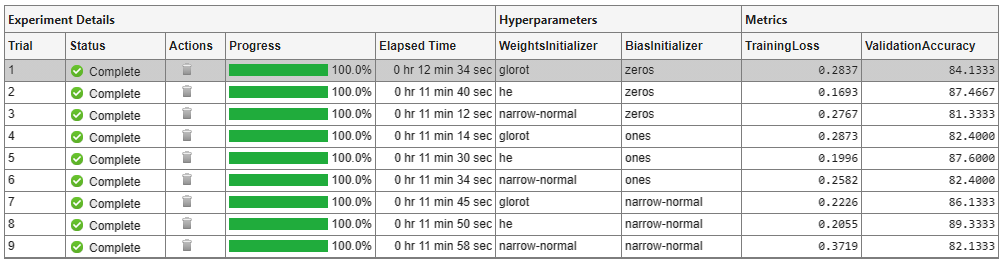To display the training plot and track the progress of each trial while the experiment is running, under Review Results, click Training Plot.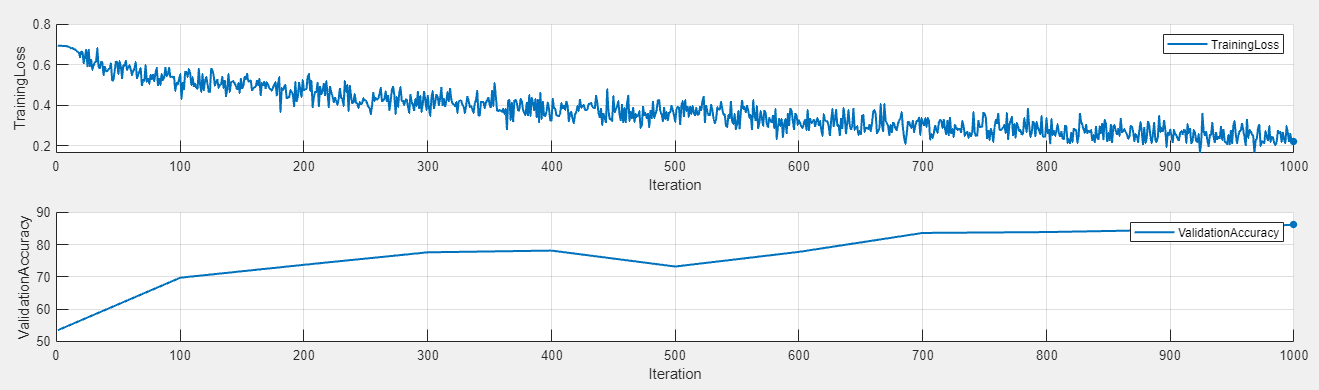### Evaluate Results

To find the best result for your experiment, sort the table of results by validation accuracy.

1. Point to the ValidationAccuracy column.

2. Click the triangle icon.

3. Select Sort in Descending Order.

The trial with the highest validation accuracy appears at the top of the results table.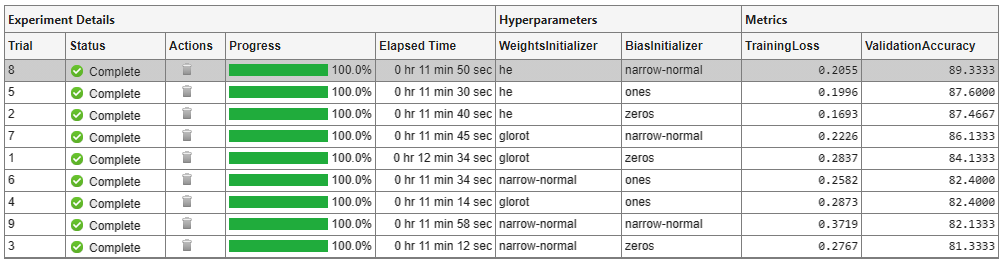To visually check that the network correctly identifies similar and dissimilar pairs, select the top row in the results table and, under Review Results, click Test Images. Experiment Manager displays ten randomly selected pairs of test images with the prediction from the trained network, the probability score, and a label indicating whether the prediction is correct or incorrect.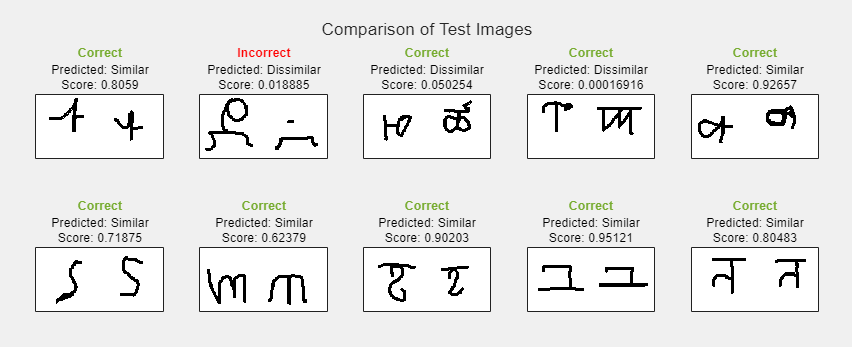To perform additional computations, export the training output to the workspace as a structure. The `trainedNet` field of this structure contains the trained network.

1. On the Experiment Manager toolstrip, click Export > Training Output.

2. In the dialog window, enter the name of a workspace variable for the exported training output. The default name is `trainingOutput`.

1. In the results table, right-click the ValidationAccuracy cell of the best trial.

3. In the Annotations pane, enter your observations in the text box.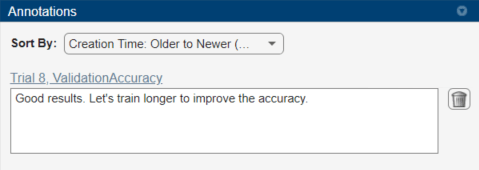### Close Experiment

In the Experiment Browser pane, right-click the name of the project and select Close Project. Experiment Manager closes all of the experiments and results contained in the project.

### Training Function

This function specifies the training data, network architecture, training options, and training procedure used by the experiment. The input to this function is a structure with fields from the hyperparameter table and an `experiments.Monitor` object that you can use to track the progress of the training, record values of the metrics used by the training, and produce training plots. The training function returns a structure that contains the trained network, the weights for the final `fullyconnect` operation for the network, and the execution environment used for training. Experiment Manager saves this output so you can export it to the MATLAB workspace when the training is complete.

```function output = ImageComparisonExperiment_training(params,monitor) ```

Initialize Output

```output.network = []; output.weights = []; output.executionEnvironment = "auto"; ```

Load and Preprocess Training and Test Data

```monitor.Status = "Loading Training Data"; url = "https://github.com/brendenlake/omniglot/raw/master/python/images_background.zip"; downloadFolder = tempdir; filename = fullfile(downloadFolder,"images_background.zip"); dataFolderTrain = fullfile(downloadFolder,"images_background"); if ~exist(dataFolderTrain,"dir") websave(filename,url); unzip(filename,downloadFolder); end imdsTrain = imageDatastore(dataFolderTrain, ... IncludeSubfolders=true, ... LabelSource="none"); files = imdsTrain.Files; parts = split(files,filesep); labels = join(parts(:,(end-2):(end-1)),"_"); imdsTrain.Labels = categorical(labels); monitor.Status = "Loading Test Data"; url = "https://github.com/brendenlake/omniglot/raw/master/python/images_evaluation.zip"; filename = fullfile(downloadFolder,"images_evaluation.zip"); dataFolderTest = fullfile(downloadFolder,"images_evaluation"); if ~exist(dataFolderTest,"dir") websave(filename,url); unzip(filename,downloadFolder); end imdsTest = imageDatastore(dataFolderTest, ... IncludeSubfolders=true, ... LabelSource="none"); files = imdsTest.Files; parts = split(files,filesep); labels = join(parts(:,(end-2):(end-1)),"_"); imdsTest.Labels = categorical(labels); ```

Define Network Architecture

```monitor.Status = "Creating Network"; layers = [ imageInputLayer([105 105 1],Normalization="none") convolution2dLayer(10,64, ... WeightsInitializer=params.WeightsInitializer, ... BiasInitializer=params.BiasInitializer) reluLayer() maxPooling2dLayer(2,Stride=2) convolution2dLayer(7,128, ... WeightsInitializer=params.WeightsInitializer, ... BiasInitializer=params.BiasInitializer) reluLayer() maxPooling2dLayer(2,Stride=2) convolution2dLayer(4,128, ... WeightsInitializer=params.WeightsInitializer, ... BiasInitializer=params.BiasInitializer) reluLayer() maxPooling2dLayer(2,Stride=2) convolution2dLayer(5,256, ... WeightsInitializer=params.WeightsInitializer, ... BiasInitializer=params.BiasInitializer) reluLayer() fullyConnectedLayer(4096, ... WeightsInitializer=params.WeightsInitializer, ... BiasInitializer=params.BiasInitializer)]; lgraph = layerGraph(layers); net = dlnetwork(lgraph); fcWeights = dlarray(0.01*randn(1,4096)); fcBias = dlarray(0.01*randn(1,1)); fcParams = struct(... "FcWeights",fcWeights,... "FcBias",fcBias); output.network = net; output.weights = fcParams; ```

Specify Training Options

```numIterations = 1000; miniBatchSize = 180; validationFrequency = 100; initialLearnRate = 6e-5; gradientDecayFactor = 0.9; squaredGradientDecayFactor = 0.99; trailingAvgSubnet = []; trailingAvgSqSubnet = []; trailingAvgParams = []; trailingAvgSqParams = []; ```

Train Model

```monitor.Metrics = ["TrainingLoss" "ValidationAccuracy"]; monitor.XLabel = "Iteration"; monitor.Status = "Training"; for iteration = 1:numIterations [X1,X2,pairLabels] = getSiameseBatch(imdsTrain,miniBatchSize); X1 = dlarray(single(X1),"SSCB"); X2 = dlarray(single(X2),"SSCB"); if (output.executionEnvironment == "auto" && canUseGPU) || ... output.executionEnvironment == "gpu" X1 = gpuArray(X1); X2 = gpuArray(X2); end [loss,gradientsSubnet,gradientsParams] = dlfeval(@modelLoss, ... net,fcParams,X1,X2,pairLabels); lossValue = double(gather(extractdata(loss))); [net,trailingAvgSubnet,trailingAvgSqSubnet] = ... adamupdate(net,gradientsSubnet, ... trailingAvgSubnet,trailingAvgSqSubnet, ... iteration,initialLearnRate,gradientDecayFactor,squaredGradientDecayFactor); [fcParams,trailingAvgParams,trailingAvgSqParams] = ... adamupdate(fcParams,gradientsParams, ... trailingAvgParams,trailingAvgSqParams, ... iteration,initialLearnRate,gradientDecayFactor,squaredGradientDecayFactor); if ~rem(iteration,validationFrequency) || iteration == 1 || iteration == numIterations monitor.Status = "Validating"; accuracy = zeros(1,5); accuracyBatchSize = 150; for i = 1:5 [XAcc1,XAcc2,pairLabelsAcc] = getSiameseBatch(imdsTest,accuracyBatchSize); XAcc1 = dlarray(single(XAcc1),"SSCB"); XAcc2 = dlarray(single(XAcc2),"SSCB"); if (output.executionEnvironment == "auto" && canUseGPU) || ... output.executionEnvironment == "gpu" XAcc1 = gpuArray(XAcc1); XAcc2 = gpuArray(XAcc2); end Y = predictSiamese(net,fcParams,XAcc1,XAcc2); Y = round(Y); accuracy(i) = sum(Y == pairLabelsAcc)/accuracyBatchSize; end recordMetrics(monitor,iteration, ... ValidationAccuracy=mean(accuracy)*100); monitor.Status = "Training"; end output.network = net; output.weights = fcParams; recordMetrics(monitor,iteration, ... TrainingLoss=lossValue); monitor.Progress = (iteration/numIterations)*100; if monitor.Stop return; end end ```

Display Pairs of Test Images

```testBatchSize = 10; [XTest1,XTest2,pairLabelsTest] = getSiameseBatch(imdsTest,testBatchSize); XTest1 = dlarray(single(XTest1),"SSCB"); XTest2 = dlarray(single(XTest2),"SSCB"); if (output.executionEnvironment == "auto" && canUseGPU) || ... output.executionEnvironment == "gpu" XTest1 = gpuArray(XTest1); XTest2 = gpuArray(XTest2); end YScore = predictSiamese(net,fcParams,XTest1,XTest2); YScore = gather(extractdata(YScore)); YPred = round(YScore); XTest1 = extractdata(XTest1); XTest2 = extractdata(XTest2); figure(Name="Test Images"); title(tiledlayout(2,5), ... "Comparison of Test Images") for i = 1:numel(pairLabelsTest) if pairLabelsTest(i) == YPred(i) titleStr = "Correct"; titleColor = "#77AC30"; % dark green else titleStr = "Incorrect"; titleColor = "#FF0000"; % red end if YPred(i) == 1 predStr = "Predicted: Similar"; else predStr = "Predicted: Dissimilar" ; end scoreStr = "Score: " + YScore(i); nexttile imshow([XTest1(:,:,:,i) XTest2(:,:,:,i)]); imageTitle = title(titleStr,[predStr,scoreStr]); imageTitle.Color = titleColor; xticks([]) yticks([]) end ```
```end ```

### Helper Functions

The `modelLoss` function takes as input the Siamese `dlnetwork` object `net`, a pair of mini-batch input data `X1` and `X2`, and the label indicating whether they are similar or dissimilar. The function returns the loss with respect to the learnable parameters in the network and the binary cross-entropy loss between the prediction and the ground truth.

```function [loss,gradientsSubnet,gradientsParams] = modelLoss(net,fcParams,X1,X2,pairLabels) Y = forwardSiamese(net,fcParams,X1,X2); loss = binarycrossentropy(Y,pairLabels); [gradientsSubnet,gradientsParams] = dlgradient(loss,net.Learnables,fcParams); end ```

The `binarycrossentropy` function returns the binary cross-entropy loss value for a prediction from the network.

```function loss = binarycrossentropy(Y,pairLabels) precision = underlyingType(Y); Y(Y < eps(precision)) = eps(precision); Y(Y > 1 - eps(precision)) = 1 - eps(precision); ```
``` loss = -pairLabels.*log(Y) - (1 - pairLabels).*log(1 - Y); loss = sum(loss)/numel(pairLabels); end```

The `forwardSiamese` function defines how the subnetworks and the `fullyconnect` and `sigmoid` operations combine to form the complete Siamese network. The function accepts the network structure and two training images and returns a prediction of the probability of the pair being similar (closer to 1) or dissimilar (closer to 0).

```function Y = forwardSiamese(net,fcParams,X1,X2) F1 = forward(net,X1); F1 = sigmoid(F1); ```
``` F2 = forward(net,X2); F2 = sigmoid(F2);```
``` Y = abs(F1 - F2); Y = fullyconnect(Y,fcParams.FcWeights,fcParams.FcBias); Y = sigmoid(Y); end```

The `getSiameseBatch` function returns a randomly selected batch of paired images. On average, this function produces a balanced set of similar and dissimilar pairs.

```function [X1,X2,pairLabels] = getSiameseBatch(imds,miniBatchSize) pairLabels = zeros(1,miniBatchSize); imgSize = size(readimage(imds,1)); X1 = zeros([imgSize 1 miniBatchSize]); X2 = zeros([imgSize 1 miniBatchSize]); for i = 1:miniBatchSize choice = rand(1); if choice < 0.5 [pairIdx1,pairIdx2,pairLabels(i)] = getSimilarPair(imds.Labels); else [pairIdx1,pairIdx2,pairLabels(i)] = getDissimilarPair(imds.Labels); end X1(:,:,:,i) = imds.readimage(pairIdx1); X2(:,:,:,i) = imds.readimage(pairIdx2); end end ```

The `getSimilarPair` function returns a random pair of indices for images that are in the same class and the similar pair label of 1.

```function [pairIdx1,pairIdx2,label] = getSimilarPair(classLabel) classes = unique(classLabel); classChoice = randi(numel(classes)); idxs = find(classLabel==classes(classChoice)); pairIdxChoice = randperm(numel(idxs),2); pairIdx1 = idxs(pairIdxChoice(1)); pairIdx2 = idxs(pairIdxChoice(2)); label = 1; end ```

The `getDissimilarPair` function returns a random pair of indices for images that are in different classes and the dissimilar pair label of 0.

```function [pairIdx1,pairIdx2,label] = getDissimilarPair(classLabel) classes = unique(classLabel); classesChoice = randperm(numel(classes),2); idxs1 = find(classLabel==classes(classesChoice(1))); idxs2 = find(classLabel==classes(classesChoice(2))); pairIdx1Choice = randi(numel(idxs1)); pairIdx2Choice = randi(numel(idxs2)); pairIdx1 = idxs1(pairIdx1Choice); pairIdx2 = idxs2(pairIdx2Choice); label = 0; end ```

The `predictSiamese` function uses the trained network to make predictions about the similarity of two images.

```function Y = predictSiamese(net,fcParams,X1,X2) F1 = predict(net,X1); F1 = sigmoid(F1); ```
``` F2 = predict(net,X2); F2 = sigmoid(F2);```
``` Y = abs(F1 - F2); Y = fullyconnect(Y,fcParams.FcWeights,fcParams.FcBias); Y = sigmoid(Y); end```